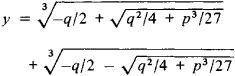# Cubic Equation

(redirected from Cardanic formulae)
Also found in: Dictionary.

## cubic equation

[′kyü·bik i′kwā·zhən]
(mathematics)
A polynomial equation with no exponent larger than 3.

## Cubic Equation

an algebraic equation of the third degree. The general form of a cubic equation is

ax3 + bx2 + cx + d = 0

where a ≠ 0. By replacing x in this equation by a new unknown y related to x by x = y − b/3a, a cubic equation can be reduced to the simpler (canonical) form

y3 + py + q = 0

where

p = b2/3a2 = c/a

q = 2b2/27a3bc/3a2 + da

The solution of this equation can be found using Cardan’s formulaIf the coefficients of a cubic equation are real, then the nature of its roots depends on the sign of the radicand q2/4 + p/27 in Cardan’s formula. If q2/4 + p3/4 + p3/27 > 0, then the cubic equation has three different roots, one real and two complex conjugates. If q2/4 + p327 = 0, then all three roots are real, two of them being equal. If q2/4 + p3/27 > 0, then the three roots are real and different. The expression q2/4 + p3/27 differs by a constant factor from the discriminant of a cubic equation D = −4p3 − 27q2.

### REFERENCES

Kurosh, A. G. Kurs vysshei algebry, 9th ed. Moscow, 1968.
Entsiklopediia elementarnoi matematiki, book 2. [Edited by P. S. Aleksandrov (et al.).] Moscow-Leningrad, 1951.
Site: Follow: Share:
Open / Close